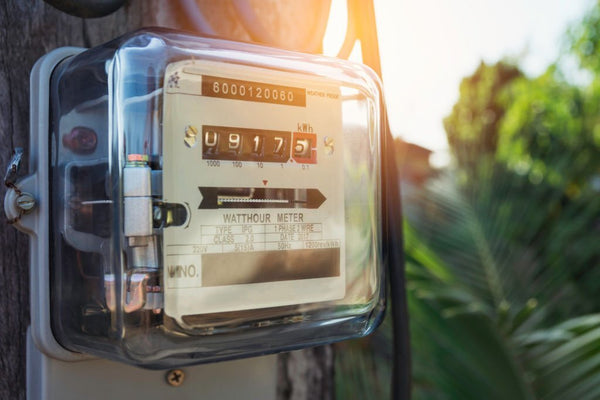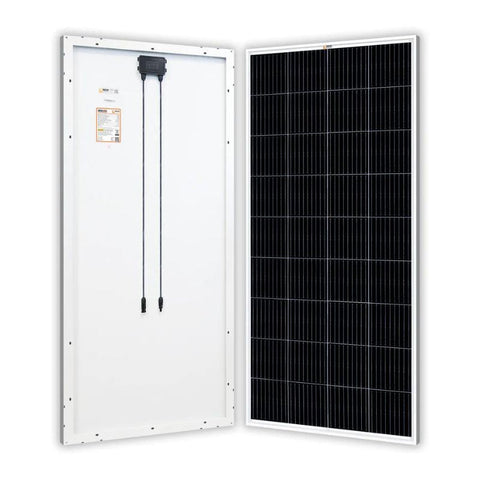Convert KWH to KVA (Electrical Conversions & Guide) - ShopSolar.com

# Convert kWh to kVA

In the world of solar and electrical power, conversion and calculations are fairly common.

We might need to change one variable to another so we have the correct variables, or we need a numerical value to understand the scale of our system.

To this end, let’s have a look at how to convert kWh to kVA.

But before we can look at the conversion, we need to understand the units as individual variables.

## What Are kWhChances are that you’ll come across a kWh calculator when starting with your solar system.

So what’s the purpose of such a calculator and what makes that unit of power important in the world of solar?

Well, to understand kilowatts and kilowatt hours, we need to distinguish between energy and power.

Once we know the difference, we’ll be able to understand how to convert kW to kWh and vice versa.

### Kilowatts

Kilowatts and watts refer to power. A kilowatt is equivalent to 1,000 watts.

In the electrical world, power is the rate that an appliance uses or produces energy.

Let’s say we have a 20 W lightbulb and a 100 W lightbulb being powered individually. You’ll notice that the 20 W lightbulb is dimmer than the 100 W lightbulb.

The reason for this is that we’re pushing electricity into the 20 W lightbulb at a lower rate than the 100 W one.

In the world of solar, we can use watts and kilowatts to measure how much electricity a solar system produces.

If you have a 3 kW solar system made up of an array of 200 W Monocrystalline Solar Panels set up on your roof. You’ll notice that around the midday period is when the solar system will peak its power output.This peak is how your system is rated.

If we graph the hours in a day and measure the solar system’s output each hour, we can use these values in a kilowatt-hour cost calculator.

This helps us size our solar system and know how much power we’re generating compared to how much power we’re using.

### Kilowatt-Hours

Kilowatt-hours refers to energy, which is the power used or produced over time.

It’s easy to confuse kW and kWh, but once we can distinguish the difference between them, we can convert kWh to kW and vice versa.

Let’s say you use a kilowatt of power for one hour. In this case, you’ve used a one-kilowatt hour of energy.

However, this is a very simple example so let’s use a more relatable one.

Many turn to the water in a hose pipe analogy. The faster the water flows through the pipe, the higher the power will be on the receiving end.

If we fill a bucket with that hose pipe, the amount of water collected in the bucket can be thought of as the amount of energy stored in a battery.

In other words, we have a house pipe filling up a bucket, and a wire charging a battery.

The bucket is sized by the amount of water it can hold, and the battery is sized by the amount of energy it can store and is measured in kWh.

## What Are kVA?

A common cause of confusion is the battle between kilowatt-hours and kilo-volt amperes.

When we speak about kVA in the electrical and solar world, we’re referring to the amount of electrical power that a meter can feed to a building or site.

A single kilovolt-ampere is equivalent to 1,000 W of power.

kVA is a measurement of the apparent power of an electrical system or circuit. kVA and kW are equivalent in DC circuits, and the voltage and current are never out of phase.

However, in AC circuits, real power, or kW, is different from perceived power.

In this instance, kW is the actual power that performs the work, whereas kVA represents only a portion of that power and the remaining amount is referred to as the current.### Calculations

You need to be aware of the circuit's voltage and current to calculate kVA, which is a straightforward formula.

The same variables are required for a VA or kW to amps calculator.

According to the formula, you can calculate kilovolt-amps by multiplying the voltage and current by the square root of 3, then dividing the result by 1,000.

Consider a circuit with a voltage of 500 V and a current flowing at 50 A for a more concrete example with values. We’re looking for the kVA of the circuit.

If we use our formula, the result will be the square root of 3, multiplied by the product of 500 and 50, all of which will be divided by 1,000. This will give us a kVA of around 43.3.

But how do we convert kWh to kVA? Is it a simple process or one that requires further calculations?

## The Conversion

Despite the conversion not being as easy as pie and requiring a few calculations, once you know the formula, you’re all set.

The first step to converting kWh to kVA is to calculate the duration of the circuit or system’s operation. Let’s say we have a solar system and we run it for 400 seconds to test it.

We always want to work with hours during this conversion so divide the 400 seconds by 3,600 to get the value in hours. This leaves us with roughly 0.11 hours, but online converters and calculators will give you the exact value.

Now that we have the time in hours, we can divide the energy quantity in kilowatt-hours by this time value.For example, if you're converting 2 kWh of energy to kVA, we take the 2 kWh and divide it by the 0.11 hours we calculated earlier.

This gives us a value of around 18.18, known as our kVA power rating.

## Conclusion

Converting kWh to kVA isn’t a simple conversion but it’s not impossible.

Converting and calculating the value of a specific unit of power is a matter of understanding their relationships and knowing formulae.

Once we understand those concepts, converting and calculating becomes second nature.

Did You Find Our Blog Helpful? Then Consider Checking: